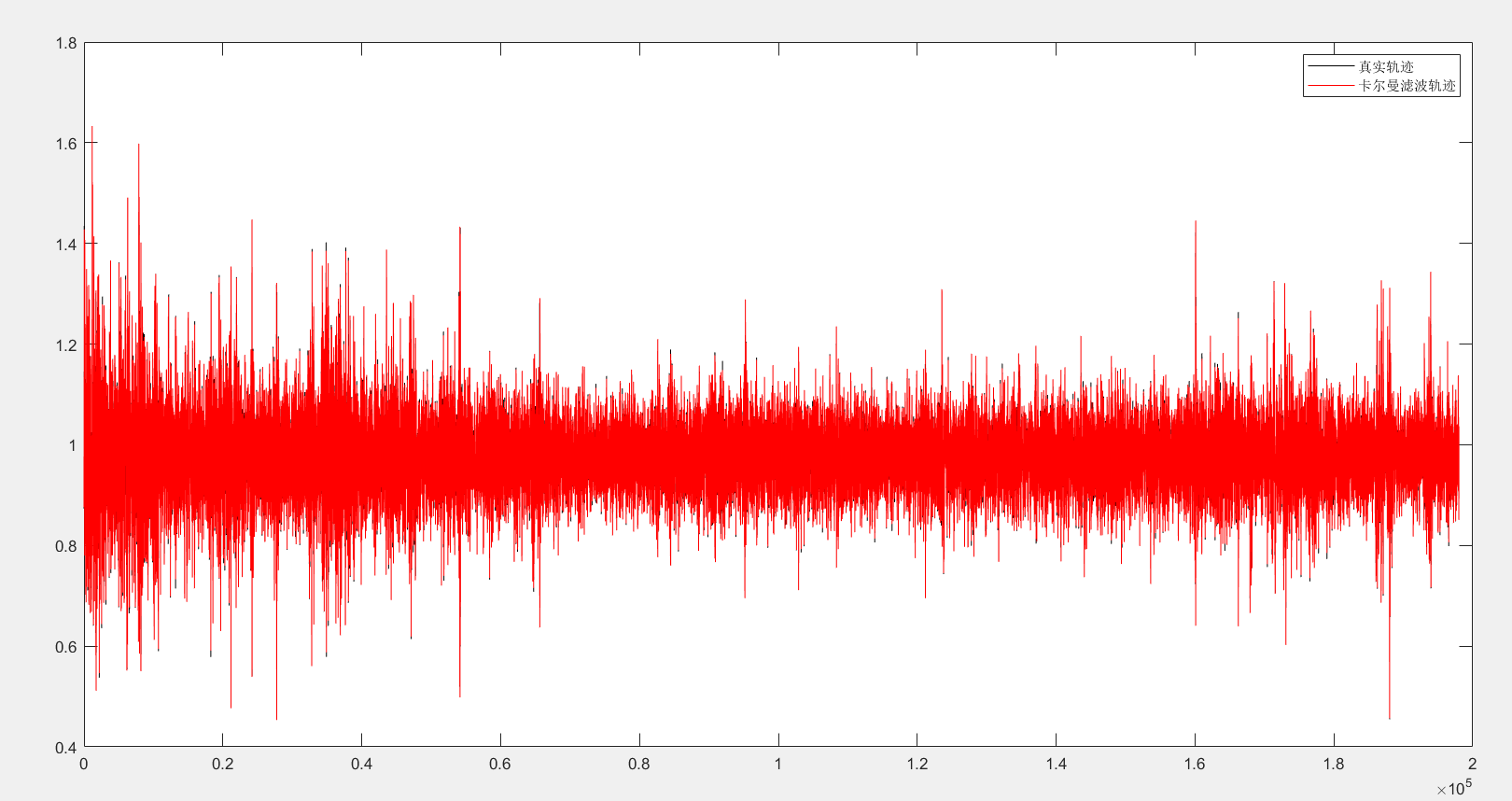2022-05-17 16:35

# 行车振动加速度的卡尔曼滤波(matlab)滤波效果不好的问题

**问题遇到的现象和发生背景 **

** 问题相关代码，请勿粘贴截图 **
（注：rdv为路面激励速度198001矩阵；acce为198001矩阵，是测量得到的加速度值）
% 状态方程：X(k+1)=FX(k)+Gw(k)
% 观测方程：Z（k）=HX(k)+v(k)
N=198000;%总时间
Q=[1 0 0 0;0 1 0 0;0 0 1 0;0 0 0 1];%过程噪声协方差
R=1e-4;%观测噪声均值
w=rdv;%随机速度作为过程噪声输入
v=sqrt(R)randn(1,N);%测量噪声V(k)
%状态方程
F = [-0.274 0.274 -5.684 0;3.824 -3.824 79.412 -700;1 -1 0 0;0 1 0 0];%状态转移矩阵
G = [0 0 0 -1]';
H = [-0.274 0.274 -5.684 0];%观测矩阵
%初始化
X=zeros(4,N);%物体真实状态
X(:,1)=[0.97;-273.44;-13.40;14.32]';%初始位移和速度
P0=[0 0 0 0;0 0 0 0;0 0 0 0;0 0 0 0];%初始误差
Z(1)=0.9511;%初始观测值
Xkf=zeros(4,N);%卡尔曼估计状态初始化
Xkf(:,1)=X(:,1);
I=eye(4); %四维系统
for k=2:N
X(:,k)=F
X(:,k-1)+Gw(k); %状态方程的驱动
Z(k)=acce(k)+v(k);%传感器对目标进行观测
%卡尔曼滤波
X_pre=F
Xkf(:,k-1);%状态预测
P_pre=FP0F'+Q;%协方差预测
Kg=P_preH'inv(HP_preH'+R);%计算卡尔曼增益
Xkf(:,k)=X_pre+Kg*(Z(k)-HX_pre);%状态更新
P0=(I-Kg
H)P_pre;%方差更新
end
y=H
Xkf
%画图
figure
hold on;box on;
plot(acce,'-k');%加速度轨迹
plot(y(1,:),'-r');%卡尔曼滤波轨迹
legend('真实轨迹','卡尔曼滤波轨迹')

**运行结果及报错内容 ****我的解答思路和尝试过的方法 **

• 写回答
• 好问题 提建议
• 追加酬金
• 关注问题
• 邀请回答